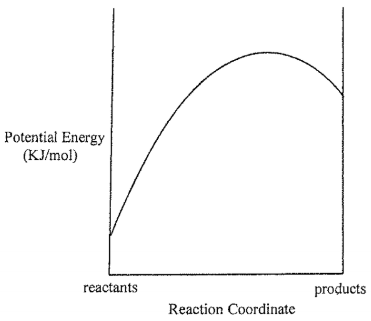# Problem: Consider the Potential Energy vs. Reaction Coordinate diagram shown to the right. For each of the following questions, circle the best answer.If a catalyst is added, i) the rate constant for the forward reaction will increase more than the rate constant for the back reactionii) the rate constant for the back reaction will increase more than the rate constant for the forward  reactioniii) both rate constants will increase to the same extentiv) the rate constant are unaffected by the addition of a catalyst

###### FREE Expert Solution

For this problem, we have to determine the effect of the addition of catalyst to rate constant of the forward and reverse reaction

• Catalysts are known to reduce the energy to reach the intermediate state in energy diagrams and hasten the reaction
• On the other hand, rate constant (k) can be calculated as:###### Problem Details

Consider the Potential Energy vs. Reaction Coordinate diagram shown to the right. For each of the following questions, circle the best answer.i) the rate constant for the forward reaction will increase more than the rate constant for the back reaction

ii) the rate constant for the back reaction will increase more than the rate constant for the forward  reaction

iii) both rate constants will increase to the same extent

iv) the rate constant are unaffected by the addition of a catalyst